## Optimum Drilling Flow Rate Example Calculation

This topic will demonstrate how to determine optimum drilling flow rate for both drilling hydraulic optimization methods (the maximum hydraulic horse power and the maximum impact force). If you don’t know the equations, please read this article first, Determine Optimum Drilling Flow Rate for Basic System – Drilling Hydraulics.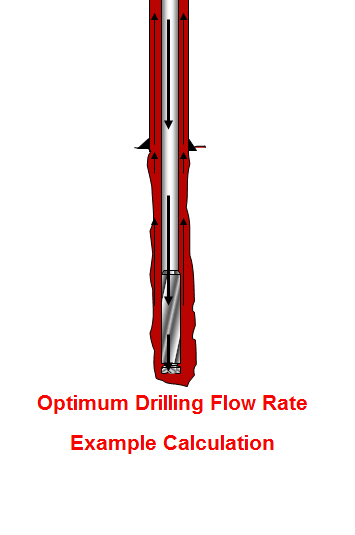The information is listed below:

Hole size = 8.5 inch

Depth In = 2,000 ft

Depth out = 6,000 ft

Mud properties at starting point

Mud weight = 9.2 ppg

PV = 13

YP = 10

## Determine Optimum Drilling Flow Rate for Basic System – Drilling Hydraulics

From the previous post (total pressure loss excluding the bit), we know the equation (shown below)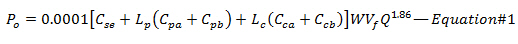We can solve the equation to get the flow rate (Q) that match with optimization criteria (Maximum Hydraulic Horsepower or Maximum Impact Force)

Maximum Hydraulic Horsepower will be happened when the pressure drop across the bit is equal to 65% of total pressure loss; therefore, 35% of total pressure loss (Ps) occurs through the drill stem (Po). The equation will be like this Po = 0.35 x Ps. By substituting Po with 0.35Ps into the Equation#1, you can solve for the optimum flow rate which will give you the maximum hydraulic horsepower.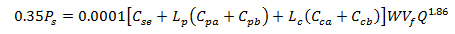Solve for Q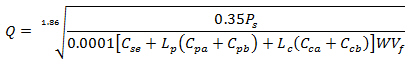Maximum Impact Force can be obtained when 48% of total pressure loss is at the bit so 52% of total pressure loss (Ps) occurs through the drill stem (Po). Substituting 52% of Ps into the Equation#1 will allow you to get the optimum flow rate at maximum impact force.Solve for Q## Total Pressure Loss through The Drilling System Without Bit

We’ve learned several calculations regarding pressure loss in the drilling system and today, we will combine all knowledge together so we will get the total pressure loss in the system excluding the bit.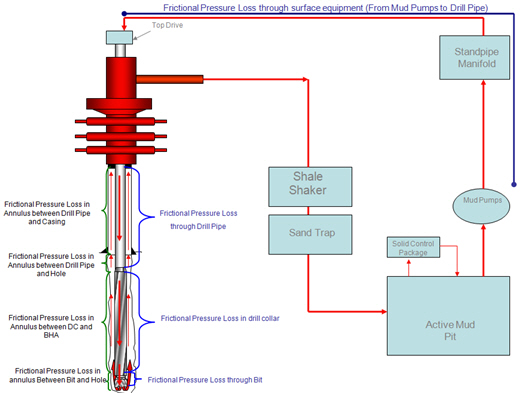Without downhole tool, the total pressure loss without bit is equal to summation of pressure loss at the surface equipment, pressure loss through drill string (drillpipe and drill collar) and pressure loss in the annulus.

The equation below demonstrates the system pressure loss excluding the bit:## Coefficient For ID of Pipe and Tool Joints

You’ve learned about pressure loss in the drill string from the previous topic (Pressure Losses through Drill String Calculation). The general coefficient for internal diameter of pipe does not account the inside diameter of tool joint.

The general coefficient equation is shown below:Coefficient of The Bore of Drill Pipe and Tool Joint

To get the accurate pressure loss calculation, the coefficient of a section of pipe must be accounted for both the pipe ID and the tool joint ID.In general, each 100 ft of pipe, there is appoximately 6.7 ft of tool joint length (6.7% of total length) and 93.3 ft of drill pipe (93.3% of total length). Therefore, the general coefficient for internal diameter of pipe can be derived in to this following equation: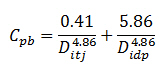## Pressure Losses Through Drill String Calculation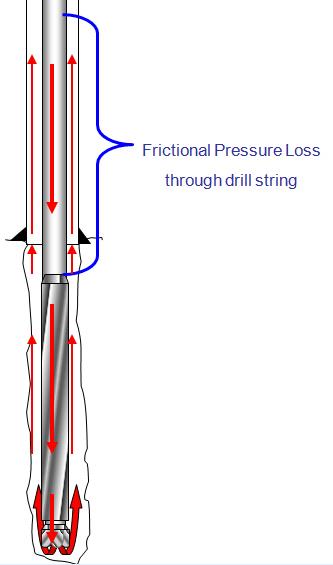To determine pressure drop while the drilling mud is passing through a circular pipe (drill pipe and drill collar), you can calculate pressure loss with the following equation: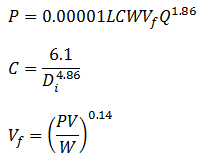Assumptions:

Turbulent viscosity = 3 cp

Friction factor = 0.378(Q/D)-0.14

The first equation has a coefficient (C) to take into account the friction and the geometry of the bore ID.

Where:

P = pressure loss, psi

L = length of pipe, ft

W = mud density, ppg

Vf = viscosity correction factor

PV = plastic viscosity, centipoises

Q = flow rate, gpm

Di = inside diameter of pipe, inch

C = general coefficient for internal diameter of pipe